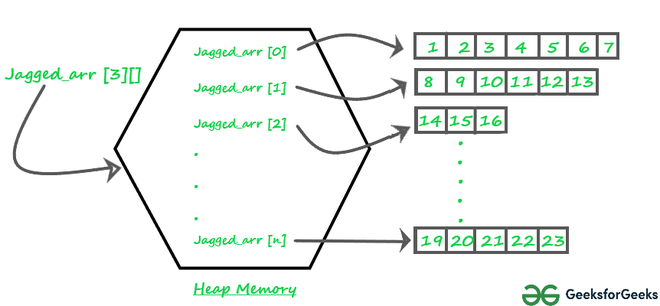GFG App
Open AppBrowser
Continue

# Jagged Array in Java

Prerequisite: Arrays in Java

A jagged array is an array of arrays such that member arrays can be of different sizes, i.e., we can create a 2-D array but with a variable number of columns in each row. These types of arrays are also known as Jagged arrays.

Pictorial representation of Jagged array in Memory:Jagged_array

Declaration and Initialization of Jagged array :

```Syntax: data_type array_name[][] = new data_type[n][];  //n: no. of rows
array_name[] = new data_type[n1] //n1= no. of columns in row-1
array_name[] = new data_type[n2] //n2= no. of columns in row-2
array_name[] = new data_type[n3] //n3= no. of columns in row-3
.
.
.
array_name[] = new data_type[nk]  //nk=no. of columns in row-n```

Alternative, ways to Initialize a Jagged array :

```                    int arr_name[][] = new int[][]  {
new int[] {10, 20, 30 ,40},
new int[] {50, 60, 70, 80, 90, 100},
new int[] {110, 120}
};

OR

int[][] arr_name = {
new int[] {10, 20, 30 ,40},
new int[] {50, 60, 70, 80, 90, 100},
new int[] {110, 120}
};

OR

int[][] arr_name = {
{10, 20, 30 ,40},
{50, 60, 70, 80, 90, 100},
{110, 120}
};```

Following are Java programs to demonstrate the above concept.

## Java

 `// Program to demonstrate 2-D jagged array in Java` `class` `Main {` `    ``public` `static` `void` `main(String[] args)` `    ``{` `        ``// Declaring 2-D array with 2 rows` `        ``int` `arr[][] = ``new` `int``[``2``][];`   `        ``// Making the above array Jagged`   `        ``// First row has 3 columns` `        ``arr[``0``] = ``new` `int``[``3``];`   `        ``// Second row has 2 columns` `        ``arr[``1``] = ``new` `int``[``2``];`   `        ``// Initializing array` `        ``int` `count = ``0``;` `        ``for` `(``int` `i = ``0``; i < arr.length; i++)` `            ``for` `(``int` `j = ``0``; j < arr[i].length; j++)` `                ``arr[i][j] = count++;`   `        ``// Displaying the values of 2D Jagged array` `        ``System.out.println(``"Contents of 2D Jagged Array"``);` `        ``for` `(``int` `i = ``0``; i < arr.length; i++) {` `            ``for` `(``int` `j = ``0``; j < arr[i].length; j++)` `                ``System.out.print(arr[i][j] + ``" "``);` `            ``System.out.println();` `        ``}` `    ``}` `}`

Output

```Contents of 2D Jagged Array
0 1 2
3 4 ```

Following is another example where i’th row has i columns, i.e., the first row has 1 element, the second row has two elements and so on.

## Java

 `// Another Java program to demonstrate 2-D jagged` `// array such that first row has 1 element, second` `// row has two elements and so on.` `class` `Main {` `    ``public` `static` `void` `main(String[] args)` `    ``{` `        ``int` `r = ``5``;`   `        ``// Declaring 2-D array with 5 rows` `        ``int` `arr[][] = ``new` `int``[r][];`   `        ``// Creating a 2D array such that first row` `        ``// has 1 element, second row has two` `        ``// elements and so on.` `        ``for` `(``int` `i = ``0``; i < arr.length; i++)` `            ``arr[i] = ``new` `int``[i + ``1``];`   `        ``// Initializing array` `        ``int` `count = ``0``;` `        ``for` `(``int` `i = ``0``; i < arr.length; i++)` `            ``for` `(``int` `j = ``0``; j < arr[i].length; j++)` `                ``arr[i][j] = count++;`   `        ``// Displaying the values of 2D Jagged array` `        ``System.out.println(``"Contents of 2D Jagged Array"``);` `        ``for` `(``int` `i = ``0``; i < arr.length; i++) {` `            ``for` `(``int` `j = ``0``; j < arr[i].length; j++)` `                ``System.out.print(arr[i][j] + ``" "``);` `            ``System.out.println();` `        ``}` `    ``}` `}`

Output

```Contents of 2D Jagged Array
0
1 2
3 4 5
6 7 8 9
10 11 12 13 14 ```

## Java

 `import` `java.util.Scanner;`   `public` `class` `JaggedArray {` `    ``public` `static` `void` `main(String[] args) {` `        ``Scanner scan = ``new` `Scanner(System.in);` `        ``System.out.print(``"Enter the number of sub-arrays: "``);` `        ``int` `numberOfArrays = scan.nextInt();` `        `  `        ``// Declare the jagged array` `        ``int``[][] jaggedArray = ``new` `int``[numberOfArrays][];` `        `  `        ``// Allocate memory to each sub-array` `        ``for` `(``int` `i = ``0``; i < numberOfArrays; i++) {` `            ``System.out.print(``"Enter the size of sub-array "` `+ (i + ``1``) + ``": "``);` `            ``int` `sizeOfSubArray = scan.nextInt();` `            ``jaggedArray[i] = ``new` `int``[sizeOfSubArray];` `        ``}` `        `  `        ``// Initialize the elements of each sub-array` `        ``for` `(``int` `i = ``0``; i < numberOfArrays; i++) {` `            ``System.out.println(``"Enter the elements of sub-array "` `+ (i + ``1``) + ``":"``);` `            ``for` `(``int` `j = ``0``; j < jaggedArray[i].length; j++) {` `                ``jaggedArray[i][j] = scan.nextInt();` `            ``}` `        ``}` `        `  `        ``// Print the elements of the jagged array` `        ``System.out.println(``"The jagged array is:"``);` `        ``for` `(``int` `i = ``0``; i < numberOfArrays; i++) {` `            ``for` `(``int` `j = ``0``; j < jaggedArray[i].length; j++) {` `                ``System.out.print(jaggedArray[i][j] + ``" "``);` `            ``}` `            ``System.out.println();` `        ``}` `        `  `        ``scan.close();` `    ``}` `}`

### Jagged arrays have the following advantages in Java:

• Dynamic allocation: Jagged arrays allow you to allocate memory dynamically, meaning that you can specify the size of each sub-array at runtime, rather than at compile-time.
• Space utilization: Jagged arrays can save memory when the size of each sub-array is not equal. In a rectangular array, all sub-arrays must have the same size, even if some of them have unused elements. With a jagged array, you can allocate just the amount of memory that you need for each sub-array.
• Flexibility: Jagged arrays can be useful when you need to store arrays of different lengths or when the number of elements in each sub-array is not known in advance.
• Improved performance: Jagged arrays can be faster than rectangular arrays for certain operations, such as accessing elements or iterating over sub-arrays, because the memory layout is more compact.

It’s important to note that jagged arrays also have some disadvantages, such as increased complexity in the code and a potentially less readable codebase, so they should be used carefully and appropriately.

This article is contributed by Rahul Agrawal. If you like GeeksforGeeks and would like to contribute, you can also write an article and mail your article to review-team@geeksforgeeks.org. See your article appearing on the GeeksforGeeks main page and help other Geeks.